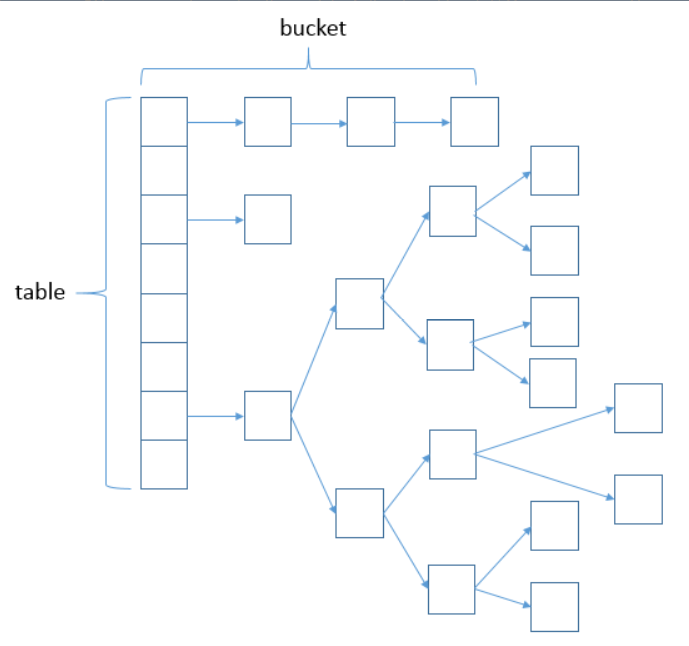# 浅谈HashMap，探索JDK（集合框架）

+关注继续查看

Collection API 位于 java.util 包中。包中的 Collection 接口是 JAVA 对于集合这一概念的抽象，存储一组类型相同的对象。

# 数据结构（数组+链表)

HashMap 是 JAVA 集合框架的成员。基于 [ 数组 + 链表 ] 的数据结构存储 key-value 形式的数据。key 是每条数据的唯一标识，HashMap 通过一个 hash 算法（也称散列算法）根据 key 值计算出这条数据在数组中的位置，即数组下标，然后把数据装载到一个链表元素Node<K, V>中，最后根据数组下标进行落桶（bucket）操作。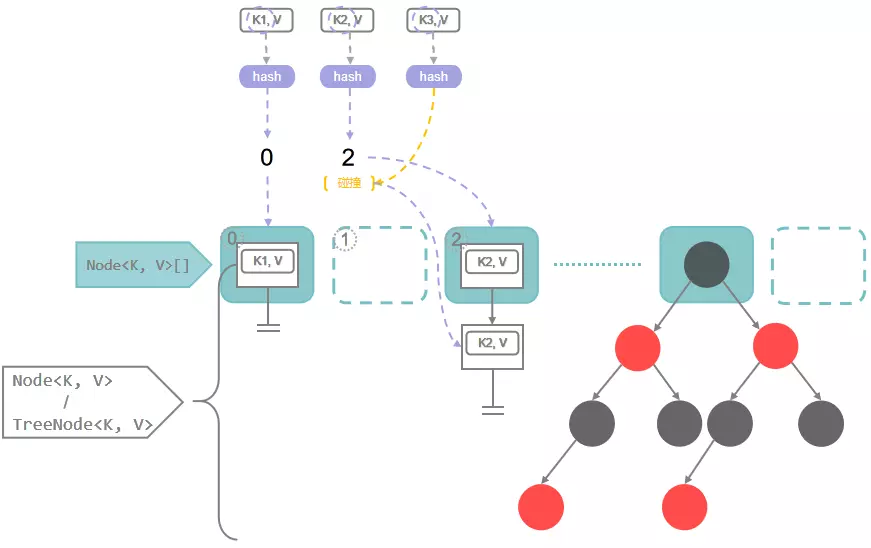# 存储(hash算法，hash冲突，初始化，扩容)

// 对用户提供的put方法
public V put(K key, V value) {
return putVal(hash(key), key, value, false, true);
}
// 散列（hash）方法
static final int hash(Object key) {
int h;
return (key == null) ? 0 : (h = key.hashCode()) ^ (h >>> 16); // 这里的位异或运算和无符号右移运算后边会详细说明
}
// 实现Map.put
// 如果对象已存在返回上一个值，如果没有则返回null
final V putVal(int hash, K key, V value, boolean onlyIfAbsent,
boolean evict) {
Node<K,V>[] tab; Node<K,V> p; int n, i;
if ((tab = table) == null || (n = tab.length) == 0) // 这里的table就是HashMap的桶数组，这个数组是需要制定容量的，默认16，属性"DEFAULT_INITIAL_CAPACITY"
n = (tab = resize()).length; // HashMap在第一次put的时候进行初始化
if ((p = tab[i = (n - 1) & hash]) == null) // 判断即将落桶的位置是否已经有Node存在，即是否存在hash冲突，这里的位与运算后边会详细说明
tab[i] = newNode(hash, key, value, null); // 不存在hash冲突直接落桶
else {
Node<K,V> e; K k;
if (p.hash == hash &&
((k = p.key) == key || (key != null && key.equals(k)))) // 判断是否在数组中存放的对象（即链表头节点）与新的对象的key值相同，如果相同直接提取到新的拷贝e中供后续操作
e = p;
else if (p instanceof TreeNode) // 如果p处于红黑树中，则调用TreeNode.putTreeVal()方法提取旧节点到e中供后续操作
e = ((TreeNode<K,V>)p).putTreeVal(this, tab, hash, key, value);
else { // 如果p处于链表中
for (int binCount = 0; ; ++binCount) { // 遍历链表
if ((e = p.next) == null) { // 当整个链表不存在与新节点相同的key，则直接把新节点加入到链表的尾部
p.next = newNode(hash, key, value, null);
if (binCount >= TREEIFY_THRESHOLD - 1) // 当链表元素数量到达指定阈值，默认8个，进行“树化”
treeifyBin(tab, hash);
break;
}
if (e.hash == hash &&
((k = e.key) == key || (key != null && key.equals(k))))
break; // 当找到与新节点相同的key，提取到e中供后续操作
p = e;
}
}
if (e != null) { // 当新节点的key已经存在（这里就是上边多处提到的“供后续操作”）
V oldValue = e.value;
if (!onlyIfAbsent || oldValue == null) // onlyIfAbsent参数的意思是“是否不覆盖旧值”
e.value = value;
return oldValue;
}
}
++modCount; // 这是一个记录操作次数的变量，后边会详细说明
if (++size > threshold) // 如果不是值覆盖会执行到这步，如果本次元素插入导致了桶数量超过阈值，则进行扩容，后边会详细说明
resize();
afterNodeInsertion(evict); // 和afterNodeAccess()方法一样，可以忽略
return null;
}
// 初始化或加倍表格大小
// 如果为null，则分配初始容量。否则，进行2次幂扩展。
final Node<K,V>[] resize() {
Node<K,V>[] oldTab = table;
int oldCap = (oldTab == null) ? 0 : oldTab.length; // 旧的数组容量
int oldThr = threshold; // 旧的扩容阈值
int newCap, newThr = 0; // 声明新的数组容量和扩容阈值
if (oldCap > 0) { // 旧的数组容量大于0说明本次是扩容操作
if (oldCap >= MAXIMUM_CAPACITY) { // 扩容前的数组大小如果已经达到最大(2^30)了
threshold = Integer.MAX_VALUE; // 修改阈值为int的最大值(2^31-1)，这样以后就不会扩容了
return oldTab;
}
else if ((newCap = oldCap << 1) < MAXIMUM_CAPACITY && // 普通扩容，在下边展开解释
oldCap >= DEFAULT_INITIAL_CAPACITY)
newThr = oldThr << 1; // 新的扩容阈值同样扩大2倍
}
else if (oldThr > 0) // 这里话多了，在下边解释
newCap = oldThr;
else { // zero initial threshold signifies using defaults, 这里和  一起看吧
newCap = DEFAULT_INITIAL_CAPACITY;
}
if (newThr == 0) { // 计算新的扩容阈值
float ft = (float)newCap * loadFactor;
newThr = (newCap < MAXIMUM_CAPACITY && ft < (float)MAXIMUM_CAPACITY ?
(int)ft : Integer.MAX_VALUE);
}
threshold = newThr;
@SuppressWarnings({"rawtypes","unchecked"})
Node<K,V>[] newTab = (Node<K,V>[])new Node[newCap];
table = newTab;
if (oldTab != null) {
// 把每个bucket都移动到新的buckets中
for (int j = 0; j < oldCap; ++j) {
Node<K,V> e;
if ((e = oldTab[j]) != null) {
oldTab[j] = null; // 删除了旧节点的引用（很细节）
if (e.next == null) // 当桶里只有一个节点，重新计算索引位置落桶
newTab[e.hash & (newCap - 1)] = e;
else if (e instanceof TreeNode)
((TreeNode<K,V>)e).split(this, newTab, j, oldCap);
else { // preserve order
// 创建两条链表
Node<K,V> loHead = null, loTail = null;
Node<K,V> hiHead = null, hiTail = null;
Node<K,V> next;
do {
next = e.next;
if ((e.hash & oldCap) == 0) { // 直接用容量与 hash 位与运算，相当于舍掉低位特征，大概是针对hash冲突进行一次随机散列。结果为0的保持原来的索引位置不变
if (loTail == null)
else
loTail.next = e;
loTail = e;
}
else { // 否则向右偏移旧数组容量
if (hiTail == null)
else
hiTail.next = e;
hiTail = e;
}
} while ((e = next) != null);
if (loTail != null) {
loTail.next = null;
}
if (hiTail != null) {
hiTail.next = null;
newTab[j + oldCap] = hiHead; // 向右偏移旧数组容量
}
}
}
}
}
return newTab;
}

# 详细说明



(h = key.hashCode()) ^ (h >>> 16)
public native int hashCode(); 



i = (n - 1) & hash
n = table.length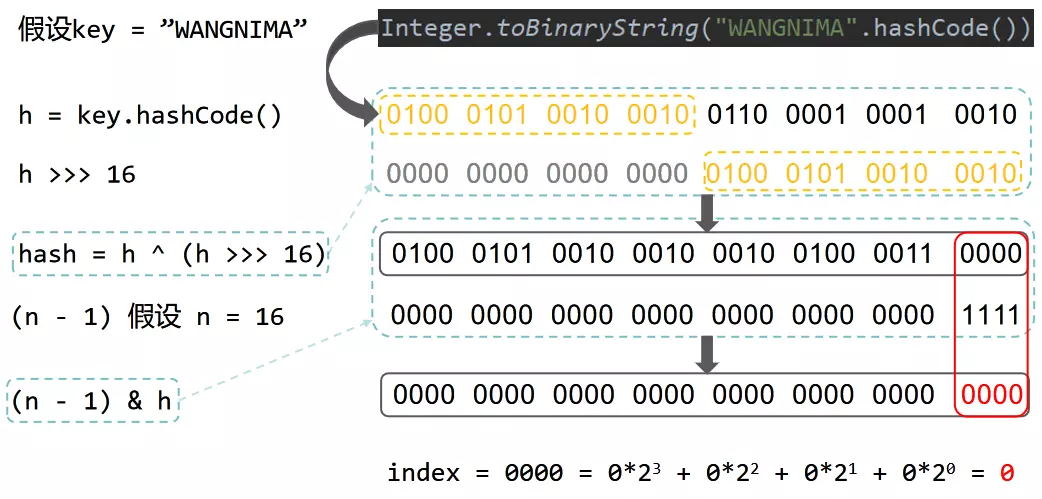1.创建一个 HashMap：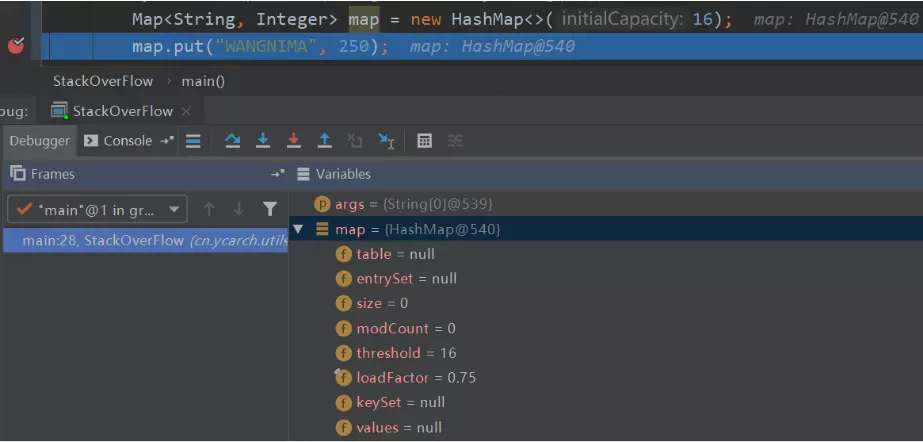2.执行下一步，put 一个 key 为 "WANGNIMA" 的元素：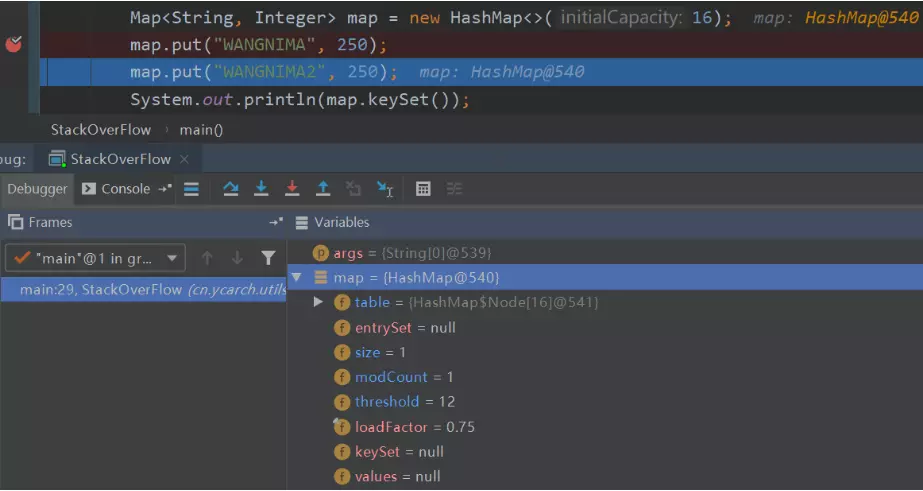3.接下来继续执行到把两个元素都 put 进去：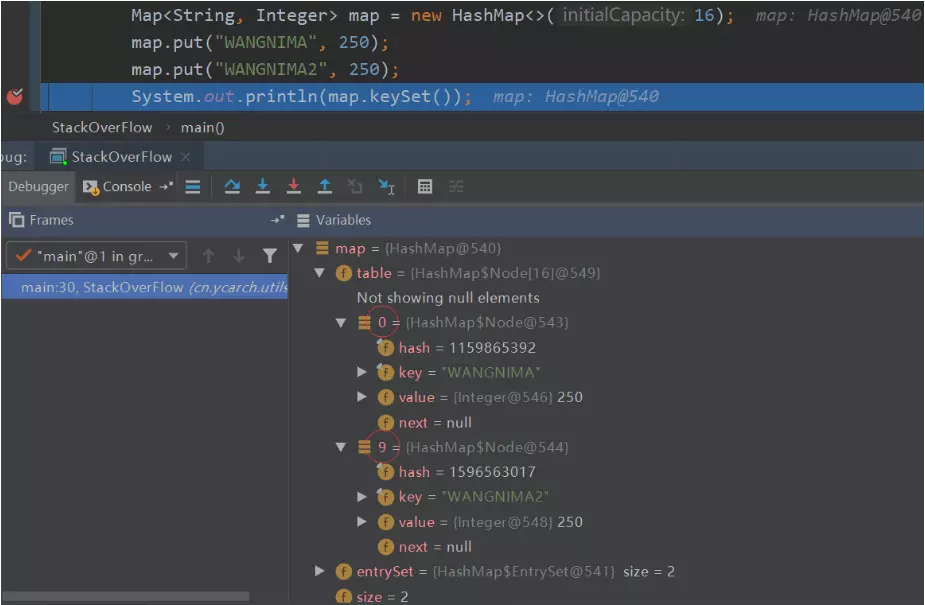4.测试 hash 冲突：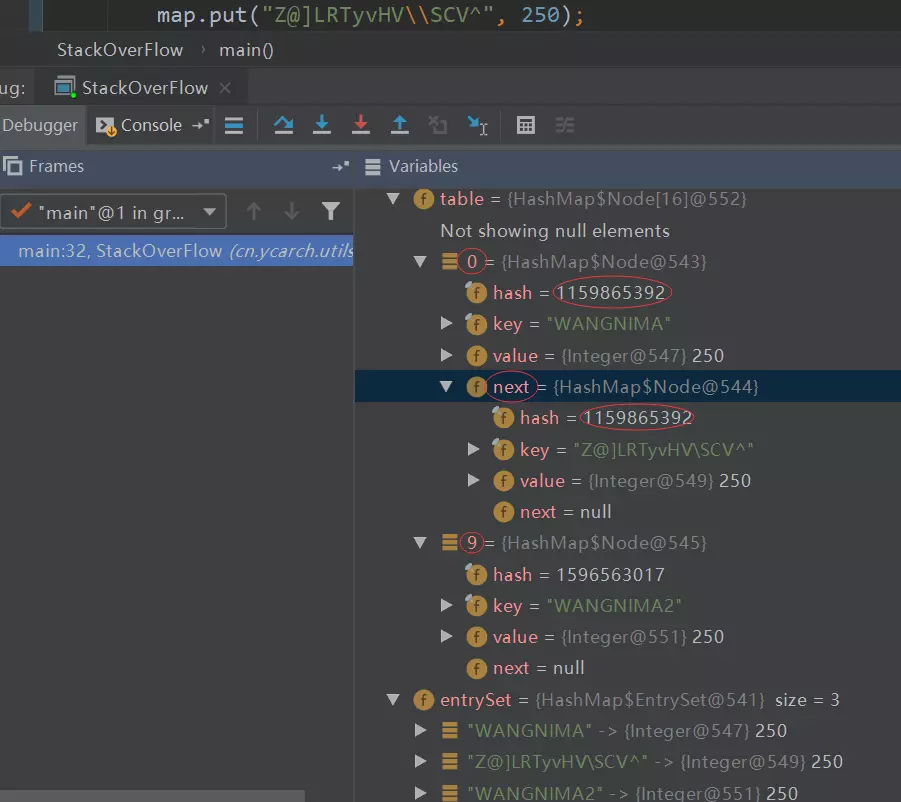++modCount;
fail-fast 机制。

    public static void main(String[] args) {
Map<String, Integer> map = new HashMap<>(16);
map.put("WANGNIMA", 250);
map.put("WANGNIMA2", 250);
for (String key : map.keySet()) {
System.out.println("Key = " + key);
}
}
// .class
public static void main(String[] args) {
Map<String, Integer> map = new HashMap(16);
map.put("WANGNIMA", 250);
map.put("WANGNIMA2", 250);
Iterator var2 = map.keySet().iterator();

while(var2.hasNext()) {
String key = (String)var2.next();
System.out.println("Key = " + key);
}

}



else if ((newCap = oldCap << 1) < MAXIMUM_CAPACITY && oldCap >= DEFAULT_INITIAL_CAPACITY)
newThr = oldThr << 1;



else if (oldThr > 0) // 旧的扩容阈值赋值给新的数组容量

newCap = oldThr;

else { // zero initial threshold signifies using defaults

newCap = DEFAULT_INITIAL_CAPACITY;

}

# 总结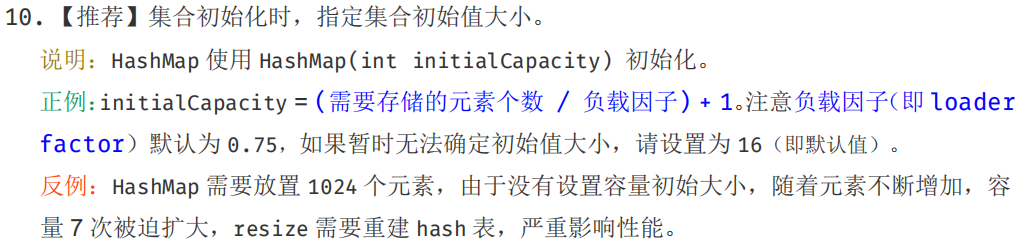|
6月前
|
Java
Java 最常见面试题：如何决定使用 HashMap 还是 TreeMap？
Java 最常见面试题：如何决定使用 HashMap 还是 TreeMap？
52 0
|

TreeMap源码分析（基于jdk1.8）

101 0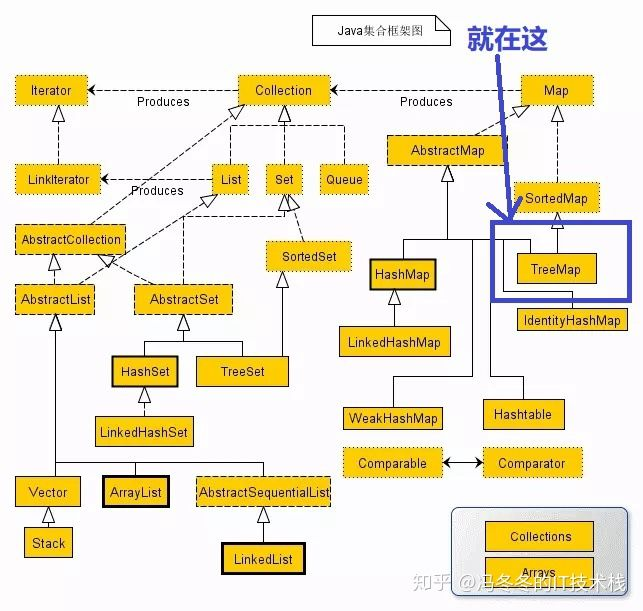|

71 0
|
Java 索引
41 0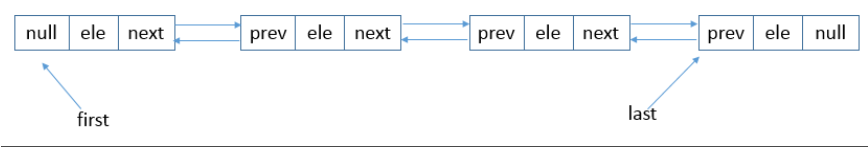|
Java 索引
【集合框架】JDK1.8源码分析之ArrayList（六）
80 0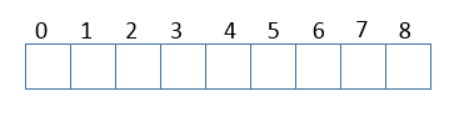【集合框架】JDK1.8源码分析之TreeMap（五）
当我们需要把插入的元素进行排序的时候，就是时候考虑TreeMap了，从名字上来看，TreeMap肯定是和树是脱不了干系的，它是一个排序了的Map,下面我们来着重分析其源码，理解其底层如何实现排序功能。下面，开始分析。
59 0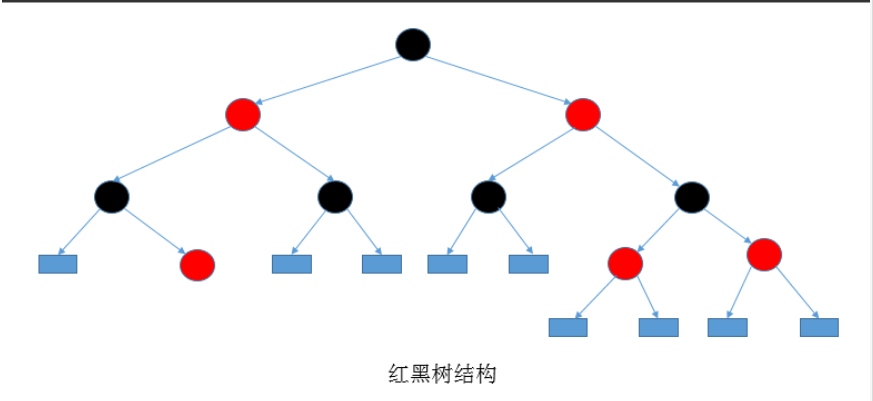74 0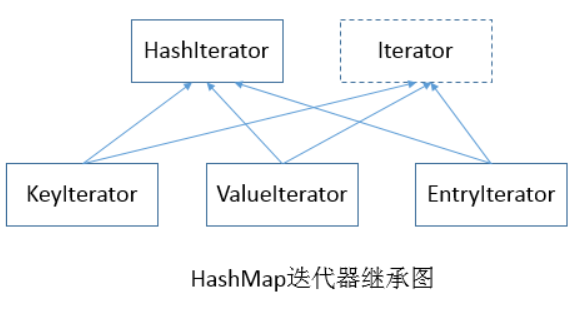|
Java API
100 0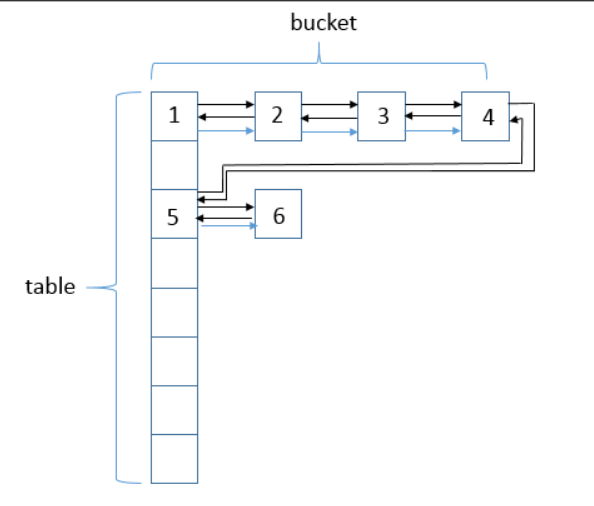|

【集合框架】JDK1.8源码分析之HashMap（一）

72 0